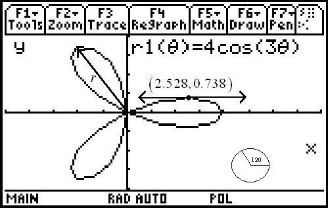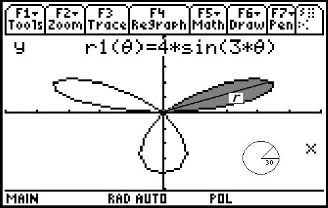# Activities

••• ##### Subject Area

• Math: Calculus: Parametric Equations and Polar Coordinates
• Math: Calculus: Applications of the Derivative
• Math: Precalculus: Polar Equations

• ##### Author9-12

15 Minutes

• ##### Device
• TI-89 / TI-89 Titanium

## Polar Necessities#### Activity Overview

Students will graphically and algebraically find the slope of the tangent line at a point on a polar graph. Finding the area of a region of a polar curve will be determined using the area formula.

#### Key Steps

•Students will explore what is necessary to understand the calculus of polar equations. They begin by reviewing polar coordinates and plotting polar graphs.

•Students will graphically and algebraically find the slope of the tangent line at a point on a polar graph.

•Finding the area of a region of a polar curve will be determined using the area formula.